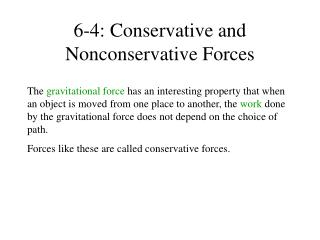DownloadDownload Presentation6-4: Conservative and Nonconservative Forces

# 6-4: Conservative and Nonconservative Forces

Télécharger la présentation## 6-4: Conservative and Nonconservative Forces

- - - - - - - - - - - - - - - - - - - - - - - - - - - E N D - - - - - - - - - - - - - - - - - - - - - - - - - - -
##### Presentation Transcript

1. 6-4: Conservative and Nonconservative Forces The gravitationalforce has an interesting property that when an object is moved from one place to another, the work done by the gravitational force does not depend on the choice of path. Forces like these are called conservative forces.

2. Definition Of A Conservative Force A force is conservative when the work it does on a moving object is independent of the path between the object's initial and final positions.

3. Definition Of A nonconservative Force A force is nonconservative when the work it does on a moving object is dependent of the path between the object's initial and final positions.

4. Examples Conservative Forces Gravitational force (Ch. 4)   Elastic spring force (Ch. 10)   Electric force (Ch. 18, 19) Nonconservative Forces Static and kinetic frictional forces   Air resistance   Tension   Normal force   Propulsion force of a rocket

5. 6.5 The Conservation of Mechanical Energy

6. THE PRINCIPLE OF CONSERVATION OF MECHANICAL ENERGY The total mechanical energy (E = KE + PE) of an object remains constant as the object moves, provided that the net work done by external nonconservative forces is zero.

7. Conservation of Mechanical Energy If friction and wind resistance are ignored, a bobsled run illustrates how kinetic and potential energy can be interconverted, while the total mechanical energy remains constant.

8. A Daredevil Motorcyclist A motorcyclist is trying to leap across the canyon shown in Figure 6.18 by driving horizontally off the cliff at a speed of 38.0 m/s. Ignoring air resistance, find the speed with which the cycle strikes the ground on the other side.

9. Roller Coaster (Ideal) The tallest and fastest roller coaster in the world is now the Steel Dragon in Mie, Japan (Figure 6.20). The ride includes a vertical drop of 93.5 m. The coaster has a speed of 3.0 m/s at the top of the drop. Neglect friction and find the speed of the riders at the bottom.

10. 6.6 Nonconservative Forces and the Work–Energy Theorem In the roller coaster example, we ignored nonconservative forces, such as friction. In reality, however, such forces are present when the roller coaster descends. The actual speed of the riders at the bottom is 41.0 m/s. Assuming again that the coaster has a speed of 3.0 m/s at the top, find the work done by nonconservative forces on a 55.0-kg rider during the descent.